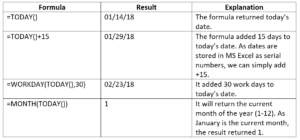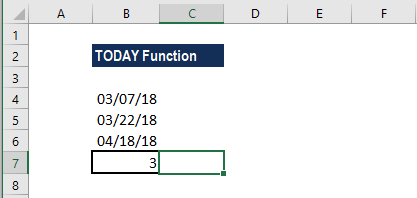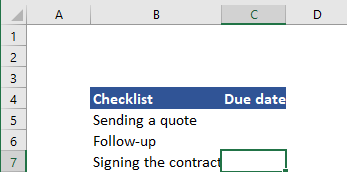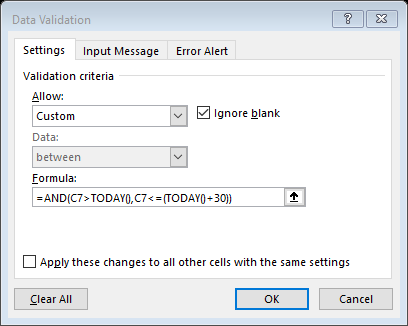# TODAY Function

Returns the current date

## What is the TODAY Function?

The TODAY function is categorized under Excel Date and Time functions. It will calculate and give the current date. It is updated continuously whenever a worksheet is changed or opened by a user. The function’s purpose is to get today’s date.

As a financial analyst, the TODAY function can be used when we wish to display the current date in a report. It is also helpful in calculating intervals. Suppose we are given a database of employees – we can use the function to calculate the age of employees as of today.

### Formula

=TODAY()

The TODAY function requires no arguments. However, it requires that you use empty parentheses ().

The function will continually update each time the worksheet is opened or recalculated, that is, each time a cell value is entered or changed. If the value doesn’t change, we need to use F9 to force the worksheet to recalculate and update the value.

However, If we need a static date, we can enter the current date using the keyboard shortcut Ctrl + Shift + ;

### How to use the TODAY Function in Excel?

The TODAY function was introduced in Excel 2007 and is available in all subsequent Excel versions. To understand the uses of the function, let us consider an example:

#### Example 1

Let’s see how the function will behave when we give the following formulas:We get the results below:When we open this worksheet on a different date, the formulas will automatically update and give a different result.

#### Example 2

Let’s now understand how we can build a data validation rule for a date using the function. Suppose we wish to create a rule that allows only a date within the next 30 days. We can use data validation with a custom formula based on the AND and TODAY functions.

Suppose we are given the following data:Different users of this file will input dates for B5, B6, and B7. We will apply data validation to C5:C7. The formula to be applied would be:If we try to input a date that is not within 30 days, we will get an error. Data validation rules are triggered when a user tries to add or change a cell value.

The TODAY function returns today’s date. It will be recalculated on an ongoing basis. The AND function takes multiple logical expressions and will return TRUE only when all expressions return TRUE. In such cases, we need to test two conditions:

1. B3>TODAY() – Checks that the date input by a user is greater than today.
2. B3<=(TODAY()+30) – Checks that the input is less than today plus 30 days.

Excel stores dates as sequential serial numbers so they can be used in calculations. By default, January 1, 1900 is serial number 1, and January 1, 2018 is serial number 43101 because it is 43,100 days after January 1, 1900. Hence, we can simply add the number of days as +30 to the TODAY function.

If both logical expressions return TRUE, the AND function returns TRUE, the data validation succeeds. If either expression returns FALSE, the validation fails.

Thanks for reading CFI’s guide to the Excel TODAY function. By taking the time to learn and master these functions, you’ll significantly speed up your financial modeling. To learn more, check out these additional CFI resources:

• Excel Functions for Finance